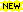# Maple tools

### Code generating with Maple - exmatCorrection: The linalg lib has to be loaded!The init_exmat procedure for Maple V R 3 users, at the bottom of the page.

### Introduction

Maple's code generating facilites are very useful. The interior details of them are interesting(?-) programming. I have used them for many projects from an engineers viewpoint, i.e. as tools that are expected to work. One of these tools are exmat, which is a procedure that generates MATLAB functions to evaluate a matrix from a set of parameters. The idea is that on some occasions you have a symbolic matrix in MAPLE, and would like MATLAB to access a numeric version of this matrix. Then exmat can generate the source code in C for a MATLAB mex function that evaluates the matrix from a vector of numerical values of the parameters. The file is ready to compile with the mex utility of MATLAB version 5. (Give the option `v4` after the 5 arguments to provide for version 4 of MATLAB, not sparse matrices though.) Along with the mex function, a short m-file that contains some help information such as in what order the parameters should be placed in the vector is written out and can be accesed with the MATLAB command help.

### Maple syntax

The Maple procedure takes 5 necessary arguments and some optional. These are

1. The name of the function to be generated, as a Maple string.
2. The file path of the function to be generated, as a Maple string.
3. The matrix.
4. A list of common subexpressions in the elements of the matrix. If you have none, give an empty list.
5. A list defining the names of the parameters and the order in which they are to be placed in the vector.
Valid options are either of these:
• The string `sparse` enclosed in backquotes. Forces exmat to generate a function that returns a sparse matrix.
• An additional list of parameter names. If present, it causes the generated function to request two input arguments with parameter values. Useful if one will use the MATLAB fzero function for example.
• The string `v4`, which causes Maple to generate the syntax for a mex-file in version 4 of MATLAB. Note that sparse matrices are not supported for MATLAB 4.

### Initialization

Before the function is useful, it should be loaded into Maple. Personally I do something like read `../exmat`;. exmat requires that some libraries are loaded. In the release 4 file these are loaded when reading the file, take a look at the top of the file itself. In the release 3 case, take a look at the init_exmat file later on this page. If you prefer to do it yourself, the libraries are:

• with(linalg):
In version V Release 3, the write library should also be loaded. Users of Maple V Release 3 should take a look at the init_exmat procedure at the bottom of this page.

### Compilation

The source code file is best compiled using the different versions of cmex or mex shell scripts that are distributed with MATLAB. I have some experience from different platforms, but these are tips only. MATLAB version 4: On a Sun use cmex CC=gcc file.c or cmex CC=acc file.c. On AIX 3.1.x use cmex CC=c89 file.c. Macintosh 68k use MPW, Power Macintosh use CodeWarrior. MATLAB version 5: On Solaris 2 use mex file.c, which can also be called from within MATLAB.

### Examples

#### Example 1a

Suppose we have a matrix M in Maple,

`M:=matrix(2,3,[a1,sin(a1),cos(a1),a2*a3,2*a2*a3,3*a2*a3]);`

Generating an evaluating function called tx could be done by

`exmat(`tx`,``,M,[],[a1,a2,a3]);`

Then, in MATLAB (version 5) the function could be compiled with

`mex tx.c`

and evaluated by

`tx([1 2 3])`

``` ans = 1.00000000000000 0.84147098480790 0.54030230586814 6.00000000000000 12.00000000000000 18.00000000000000 ```

#### Example 1b

The common subexpression `a2*a3` is present in three of the matrix elements. If this was more complex, one would want to keep it separate. Then the matrix would be

`M2:=matrix(2,3,[a1,sin(a1),cos(a1),sx1,2*sx1,3*sx1]);`

and the call to generate the function could be

`exmat(`tx2`,``,M2,[sx1=a2*a3],[a1,a2,a3]);`

#### Example 2

Secondly lets look at sparse matrices and the optional second parameter vector. Suppose we have a matrix M3 in Maple,

`M3:=matrix(2,3,[0,sin(a1),cos(a1),a2,0,0]);`

We could then generate a sparse version of this with

`exmat(`tx3`,``,M3,[],[a1],`sparse`,[a2]);`

We have also separated the two parameters necessary to evaluate the matrix. After compilation we can have MATLAB evaluate it as

`tx3(1,2)`

which gives

``` ans = (2,1) 2.00000000000000 (1,2) 0.84147098480790 (1,3) 0.54030230586814 ```

The full matrix would look like

``` full(ans) = 0.00000000000000 0.84147098480790 0.54030230586814 2.00000000000000 0.00000000000000 0.00000000000000 ```

#### Example 3

Another use is to find numerical solutions to nonlinear, and also linear 8-), equations. Lets say we want to solve sin(rho/L) numerically. We can then make a matrix with this component:

``` mx:=matrix(1,1,[sin(rho/L)]): ```

and export a function with

``` exmat(`tx`,``,mx,[],[rho],[L]); ```

After compilation we can solve this in MATLAB for the parameter value L=1.1 by

``` fzero('tx',3.3,[],[],1.1) ans = 3.45575191894877 ```

The case with no parameters is even simpler.

Further the matrix argument to exmat can be in two other forms, where sparse matrices are generated whether or not the `sparse` option is given. A tridiagonal matrix can be represented by a list of three lists of components, as in [[L1,L2,L3],[D1,D2,D3,D4],[U1,U2,U3]]. Using this nested list as the matrix argument of exmat would force it to generate a function that returns the sparse version of the following matrix

``` D1 U1 0. 0. L1 D2 U2 0. 0. L2 D3 U3 0. 0. L3 D4 ```

Another valid representation of a matrix is a list of three lists where the first list are row indices, the second list column indices, and the third the corresponding matrix elements. An example is [[1,1,2],[1,2,2],[e1,e2,e3]] which represents the sparse version of the matrix

``` e1 e2 0. e3 ```